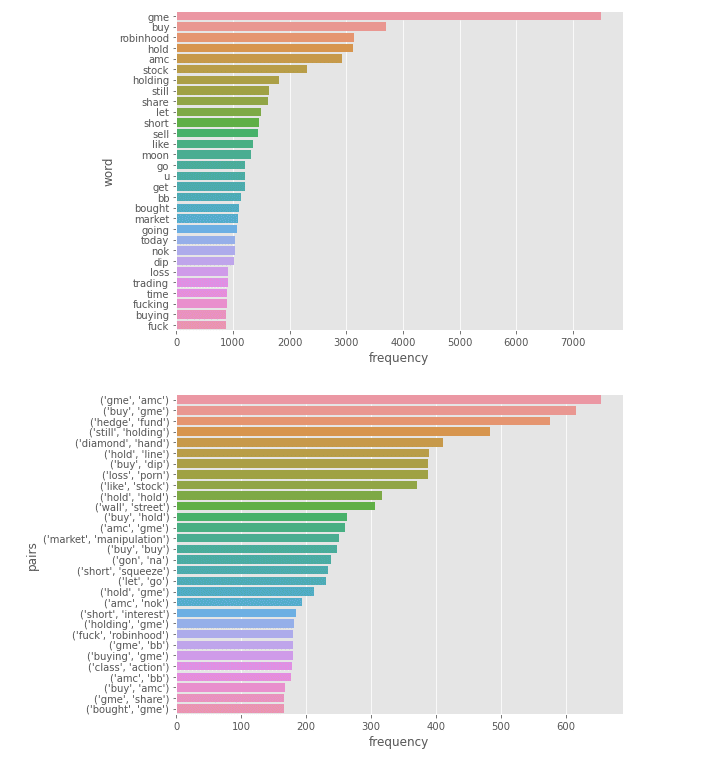# Word Frequency with Python

One of the key steps in NLP or Natural Language Process is the ability to count the frequency of the terms used in a text document or table. To achieve this we must tokenize the words so that they represent individual objects that can be counted. There are a great set of libraries that you can use to tokenize words. However, the most popular Python library is NLTK or Natural Language Tool Kit.

This tutorial will show you have to leverage NLTK to create word frequency counts and use these to create a word cloud. Let’s review the code below or watch the video presentation.

``````# loading in all the essentials for data manipulation
import pandas as pd
import numpy as np
#load in the NTLK stopwords to remove articles, preposition and other words that are not actionable
from nltk.corpus import stopwords
# This allows to create individual objects from a bog of words
from nltk.tokenize import word_tokenize
# Lemmatizer helps to reduce words to the base formfrom nltk.stem import WordNetLemmatizer
# Ngrams allows to group words in common pairs or trigrams..etc
from nltk import ngrams
# We can use counter to count the objects from collections
import Counter
# This is our visual library
import seaborn as sns
import matplotlib.pyplot as plt``````

Now that you have the basic libraries. You can review the function below that cleans the text, lowers, removes numbers, and creates data frames for word counts

``````def word_frequency(sentence):
# joins all the sentenses
sentence =” “.join(sentence)
# creates tokens, creates lower class, removes numbers and lemmatizes the words
new_tokens = word_tokenize(sentence)
new_tokens = [t.lower() for t in new_tokens]
new_tokens =[t for t in new_tokens if t not in stopwords.words(‘english’)]
new_tokens = [t for t in new_tokens if t.isalpha()]
lemmatizer = WordNetLemmatizer()
new_tokens =[lemmatizer.lemmatize(t) for t in new_tokens]
#counts the words, pairs and trigrams
counted = Counter(new_tokens)
counted_2= Counter(ngrams(new_tokens,2))
counted_3= Counter(ngrams(new_tokens,3))
#creates 3 data frames and returns thems
word_freq = pd.DataFrame(counted.items(),columns=[‘word’,’frequency’]).sort_values(by=’frequency’,ascending=False)
word_pairs =pd.DataFrame(counted_2.items(),columns=[‘pairs’,’frequency’]).sort_values(by=’frequency’,ascending=False)
trigrams =pd.DataFrame(counted_3.items(),columns=[‘trigrams’,’frequency’]).sort_values(by=’frequency’,ascending=False)
return word_freq,word_pairs,trigrams``````

The next step would be to visualize these words so that you can see how they stack up in terms of frequency.

``````# create subplot of the different data frames
fig, axes = plt.subplots(3,1,figsize=(8,20))#### Watch the Full Video Presentation of NLTK Word Frequency with WallstreetBets Data

• in
• |
• March 13, 2021#### Gaelim Holland

Subscribe
Notify of